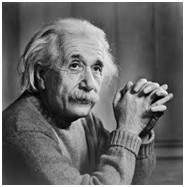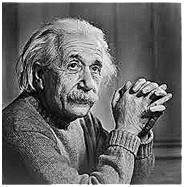# Concept of Edge Detection

We have discussed briefly about edge detection in our tutorial of introduction to masks. We will formally discuss edge detection here.

## What are edges

We can also say that sudden changes of discontinuities in an image are called as edges. Significant transitions in an image are called as edges.

### Types of edges

Generally edges are of three types:

• Horizontal edges
• Vertical Edges
• Diagonal Edges

### Why detect edges

Most of the shape information of an image is enclosed in edges. So first we detect these edges in an image and by using these filters and then by enhancing those areas of image which contains edges, sharpness of the image will increase and image will become clearer.

Here are some of the masks for edge detection that we will discuss in the upcoming tutorials.

• Prewitt Operator
• Sobel Operator
• Laplacian Operator.

Above mentioned all the filters are Linear filters or smoothing filters.

### Prewitt Operator

Prewitt operator is used for detecting edges horizontally and vertically.

### Sobel Operator

The sobel operator is very similar to Prewitt operator. It is also a derivate mask and is used for edge detection. It also calculates edges in both horizontal and vertical direction.

This operator is also known as direction mask. In this operator we take one mask and rotate it in all the 8 compass major directions to calculate edges of each direction.

Kirsch Compass Mask is also a derivative mask which is used for finding edges. Kirsch mask is also used for calculating edges in all the directions.

### Laplacian Operator

Laplacian Operator is also a derivative operator which is used to find edges in an image. Laplacian is a second order derivative mask. It can be further divided into positive laplacian and negative laplacian.

All these masks find edges. Some find horizontally and vertically, some find in one direction only and some find in all the directions. The next concept that comes after this is sharpening which can be done once the edges are extracted from the image

## Sharpening

Sharpening is opposite to the blurring. In blurring, we reduce the edge content and in Sharpening, we increase the edge content. So in order to increase the edge content in an image, we have to find edges first.

Edges can be find by one of the any method described above by using any operator. After finding edges, we will add those edges on an image and thus the image would have more edges, and it would look sharpen.

This is one way of sharpening an image.

The sharpen image is shown below.

### Original Image### Sharpen Image